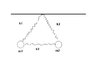# Lagrange equation (2 masses, 3 springs)

• Lindsayyyy
In summary, Lindsay found the following for the kinetic and potential energy: T=\frac {1} {2} m_{1} \dot{\vec r_{1}}^{2} + \frac {1} {2} m_{2} \dot{\vec r_{2}}^{2} V=\frac 1 2 k_{1}{\vec r_{1}}^{2}+\frac 1 2 k_{2} {\vec r_{2}}^{2}+m_{1}gy_{1}+m_{2}gy_{2}+\frac 1 2 k_{3}({\vec r_{1}}-{\vec r_{2}})^{2}

Hi

## Homework Statement

Look at the drawing. Furthermore I have a constant acceleration $$\vec g = -g \hat y$$

I shall find the Lagrange function and find the equation of motion afterwards.

## Homework Equations

Lagrange/ Euler function and eqauation

## The Attempt at a Solution

I found out the following for the kinetic energy and the potential energy:

$$T=\frac {1} {2} m_{1} \dot{\vec r_{1}}^{2} + \frac {1} {2} m_{2} \dot{\vec r_{2}}^{2}$$

and for the potential energy:

$$V=\frac 1 2 k_{1}{\vec r_{1}}^{2}+\frac 1 2 k_{2} {\vec r_{2}}^{2}+m_{1}gy_{1}+m_{2}gy_{2}+\frac 1 2 k_{3}({\vec r_{1}}-{\vec r_{2}})^{2}$$

Now I used the Euler- Lagrange equation of motion and found out that:

$$m_{1}\ddot{\vec r_{1}}=-k_{1}{\vec r_{1}}+k_{3}{\vec r_{1}}-m_{1}g\hat y$$

and
$$m_{2}\ddot{\vec r_{2}}=-k_{2}{\vec r_{2}}-k_{3}{\vec r_{2}}-m_{2}g\hat y$$

Can anyone confirm this? Or did I do any mistakes?

#### Attachments

•97.JPG
7.2 KB · Views: 509
Hi Lindsayyyy!You did not take the derivative of the k3 term correctly.

Btw, is it given that the springs have length zero in equilibrium?

yes, sorry. I forgot to type that, length zero in equilibrium is right. I trying to derive it correctly tomorrow, as I have to go out now. Thanks for help so far.

edit: It should be r2-r1 in my first post at k3. I don't know if that makes my solution right then. Am I only wrong concerning the algebraic signs? If not, I have to do it tomorrow, I'm not able to this qucik now ^^

By coincidence I noticed your edit.

Yes, it should be r2-r1 in both equations, which leaves which sign it should have exactly.
With that correction your solution is right.

Ok, thank you very much. To sum them in up to one differential equation, am I just allowed to add up both? Because I want to find the stationary points (I don't know if that's the right translation). I guess it means I have to find the points where the system is in equilibrium. I haven't tried it yet, but I would solve the differential equation, derive it two times to find the acceleration and find the zeros of the function.

I don't understand you.

Effectively you have 4 equations (one for each of x1, y1, x2, y2).
In equilibrium each acceleration would be zero, meaning each equation equals zero.
Solve the set of equations to find the coordinates for equilibrium.
No derivatives necessary.

Hm. The task say I shall find the equation of motion (so I guessed it only should be one). But I still have two ?! ^^ And I was wondering if I just add/subduct them to get "the one" equation of motion.

No, you should not add or subtract them.
I suspect they intended the equations of motion (plural).

If you really want one equation, you can define for instance:
$$\vec r = (x1, y1, x2, y2)$$
and express the equation using that.

In effect you would still have 4 equations (1 for each coordinate).

Thanks again:)

I hope you'are right they made a mistake ^^ because I have no idea if they really just want one equation